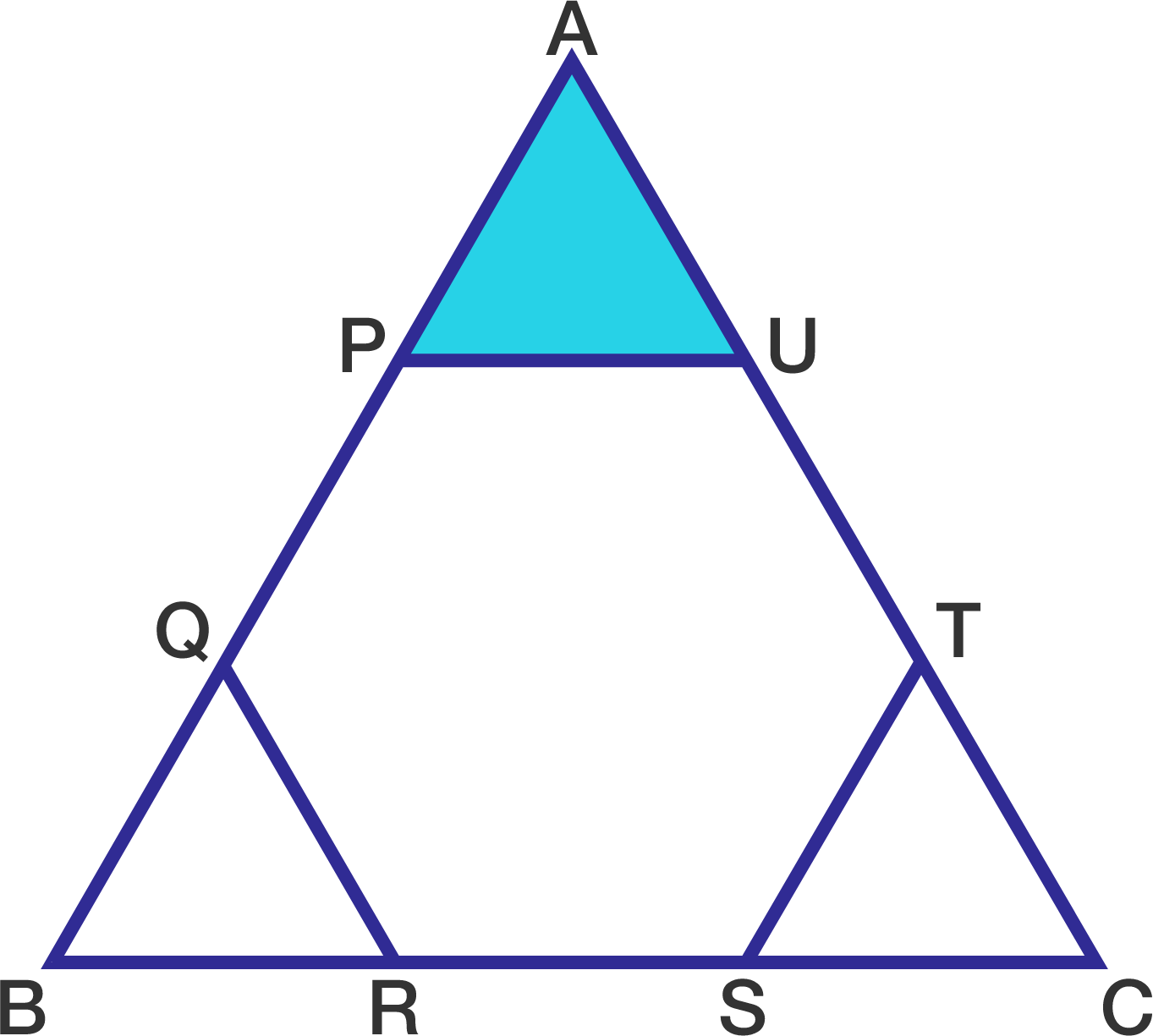# Find the shaded area

Geometry Level 1In the figure to the right, $PQRSTU$ is a regular hexagon.

Sides $PQ, RS,$ and $TU$ are extended to form triangle $ABC$.

If the area of triangle $ABC$ is 90, then find the area of the shaded region.

×

Problem Loading...

Note Loading...

Set Loading...